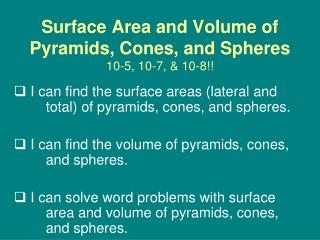# Surface Area and Volume of Pyramids, Cones, and Spheres 10-5, 10-7, & 10-8!! - PowerPoint PPT PresentationDownload PresentationSurface Area and Volume of Pyramids, Cones, and Spheres 10-5, 10-7, & 10-8!!

Surface Area and Volume of Pyramids, Cones, and Spheres 10-5, 10-7, & 10-8!!
Download Presentation## Surface Area and Volume of Pyramids, Cones, and Spheres 10-5, 10-7, & 10-8!!

- - - - - - - - - - - - - - - - - - - - - - - - - - - E N D - - - - - - - - - - - - - - - - - - - - - - - - - - -
##### Presentation Transcript

1. Surface Area and Volume of Pyramids, Cones, and Spheres10-5, 10-7, & 10-8!! I can find the surface areas (lateral and total) of pyramids, cones, and spheres. I can find the volume of pyramids, cones, and spheres. I can solve word problems with surface area and volume of pyramids, cones, and spheres.

2. Pyramids The lateral faces of a regular pyramid can be arranged to cover half of a rectangle with a height equal to the slant height of the pyramid. The width of the rectangle is equal to the base perimeter of the pyramid.

3. Pyramids SA = l + B Lateral Surface Area: LSA = l Total Surface Area: l SA = LSA + B or

4. Example SA = l + B LSA = l Find the lateral area and surface area of the regular pyramid. Step 1 Find the base perimeter and apothem. P = 6(10) = 60 in. a = Step 3 Find the surface area. Step 2 Find the lateral area.

5. Pyramids Volume: V = h

6. Example Find the volumeofthe regular hexagonal pyramid with height equal to the apothem of the base Step 1 Find the area of the base. 2 Step 2 Use the base area and the height to find the volume. = 1296 ft3

7. Cones

8. Cones SA = l + Lateral Surface Area: LSA = l Total Surface Area: l SA = LSA + B or

9. Example Find the lateral area and surface area of the cone. LSA = rℓ = (8)(17) = 136 in2 SA = rℓ+ r2 = 136 + (8)2 = 200 in2

10. Cones Volume: V = or h V =

11. Example Find the volume of a cone. Step 1 Use the Pythagorean Theorem to find the height. 162 + h2 = 342 h2 = 900 h = 30 Step 2 Use the radius and height to find the volume. = 2560 cm3

12. Example Find the volume of a conewith base circumference 25 in. and a height 2 in. more than twice the radius. Step 1 Use the circumference to find the radius. 2r = 25 r = 12.5 Step 2 Use the radius to find the height. h = 2(12.5) + 2 = 27 in. Step 3 Use the radius and height to find the volume. = 1406.25 in3

13. Spheres

14. Spheres

15. Example Find the surface area and volume of the sphere. S = 4r2 S = 4(1)2 S = 4 m2

16. Example Find the surface area and volume of the hemisphere. S = 2r2 + r2 = 2(11)2 +(11)2 = 363 in2

17. Example Find the surface area and volume of a sphere with a great circle that has an area of 49 mi2. A = r2 49 = r2 r = 7 S = 4r2 = 4(7)2 = 196 mi2

18. Example

19. Example